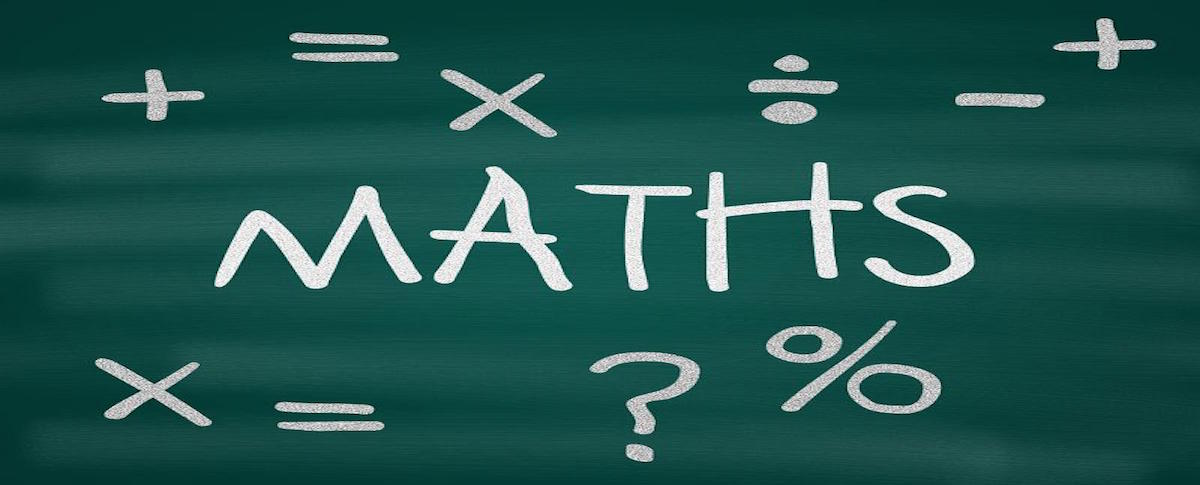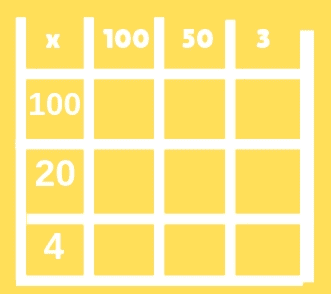# HOW TO….MATHS

I am really excited to be starting a new How to… series, starting with How to…Maths, to show and guide you through what children are being taught in schools. My hope is for you to develop the skills, knowledge and confidence to support your children with their learning at home.

As a teacher I have seen an increasing number of parents struggle to help their children with homework due to a number of factors including a lack of understanding of what is being taught as part of the National Curriculum.

This series will focus Maths and will take you through the skills, knowledge and different calculation methods used throughout Primary School from Reception to Year 6.

I have chosen to start with a method used in Year 4 – 5 to teach to multiply –The Grid Method. This method is used as a stepping-stone to the long multiplication method – which I will cover in my next blog post.

### The Grid Method in 4 StepsStep 1.

Partition the Numbers of your Multiplication Sum. What do I mean by partition? It simply means to split up the numbers. Lets take  23 x 16 as an example. The 2 in 23 represents number 20 and the 3 represents 3. Number 23 therefore is partitioned into 20 and 3. The number 16 is partitioned into 10 and 6. This is the first step to breaking down big multiplication sums. It helps children understand how numbers are made up and what each digit represents. A three-digit number would be partitioned as follows: 123 = 100, 20 and 3.

Step 2.

Set out the Grid. Using your partitioned numbers set out the grid as per step 2 in the diagram. Make sure it is clear.

Step 3.

Do the multiplication. Start by multiplying 10 x 20 and place the answer, 200 in the grid as shown. Then do 3 x 20, 20 x 6 and 3 x 6. Once your answers are placed in the grid add the columns together. Make sure the numbers are lined up correctly to avoid confusion: 200 + 120 = 320 and 30 + 18 = 48.

Step 4

The Final Stage. The last part of this method is to add 320 + 48 = 368.

23 x 16 = 368

I have shown you an example using 2 digit numbers. To multiply 3 digit numbers such as 167 x 34 or 153 x 124 the process is the same. The numbers will have to be partitioned accordingly and the grid will have to be adapted.The grid setup for 3 digit numbers.                                                                                   The calculation method remains the same.

I hope you have found the first of my How to…Maths Blog useful and will use it when helping your children with Maths. If there is anything you don’t understand and would like me to cover in my blog I would love to hear from you. Be sure to like us on Facebook and follow us on Twitter and Instagram.

If your child struggles with Maths and you are considering Private Tutoring please do not hesitate to get in touch.

This site uses Akismet to reduce spam. Learn how your comment data is processed.